Type
Quiz
Book Title
Fundamentals of Corporate Finance Standard Edition 9th Edition
ISBN 13
978-0073382395

### 978-0073382395 Chapter 6 Questions and Problems 53-66

April 3, 2019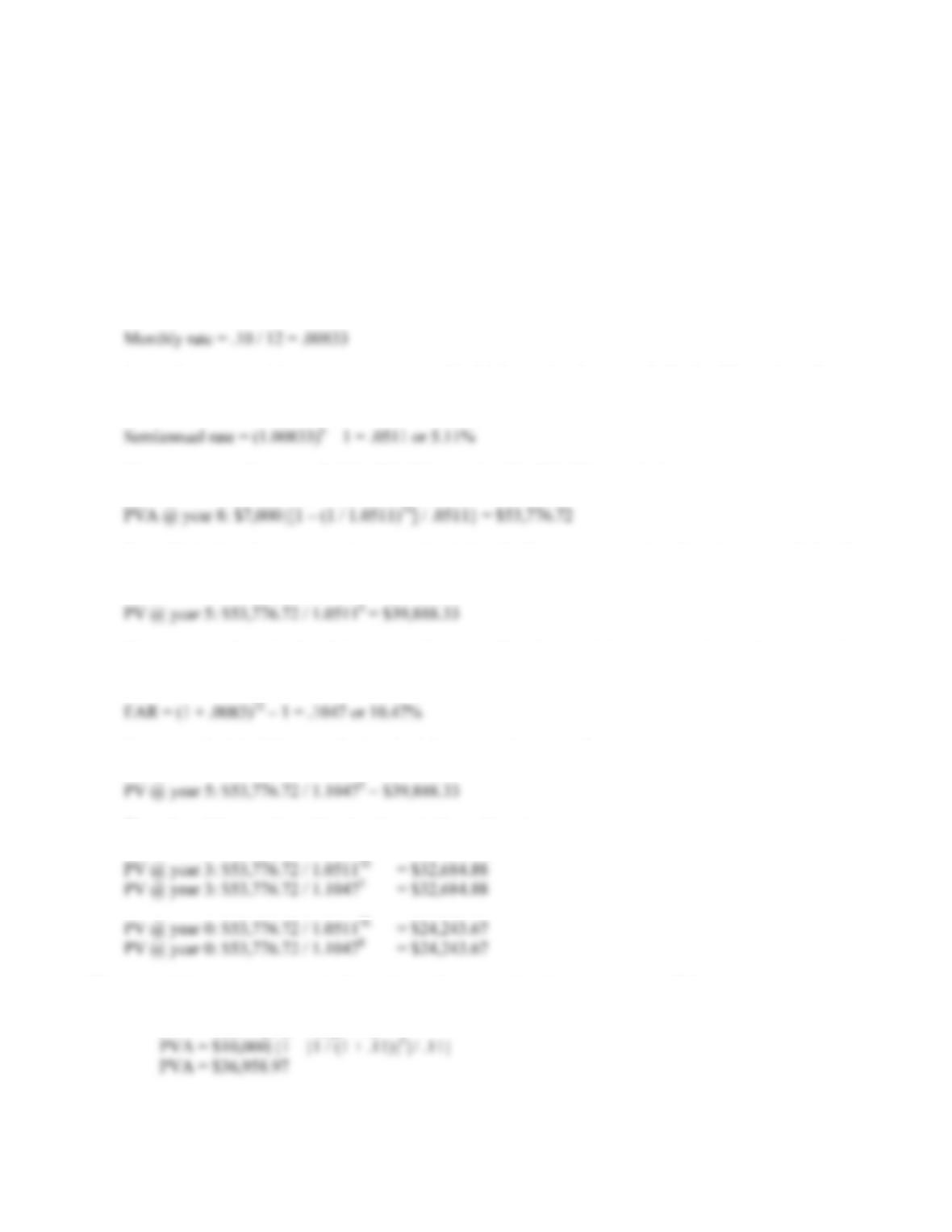B-84 SOLUTIONS
So the APR is:
APR = 12(2.361%) = 28.33%
And the EAR is:
EAR = (1.02361)12 – 1 = .3231 or 32.31%
52. The cash flows in this problem are semiannual, so we need the effective semiannual rate. The
interest rate given is the APR, so the monthly interest rate is:
To get the semiannual interest rate, we can use the EAR equation, but instead of using 12 months as the
exponent, we will use 6 months. The effective semiannual rate is:
We can now use this rate to find the PV of the annuity. The PV of the annuity is:
Note, this is the value one period (six months) before the first payment, so it is the value at year 8. So, the
value at the various times the questions asked for uses this value 8 years from now.
Note, you can also calculate this present value (as well as the remaining present values) using the number
of years. To do this, you need the EAR. The EAR is:
So, we can find the PV at year 5 using the following method as well:
The value of the annuity at the other times in the problem is:
53. a. If the payments are in the form of an ordinary annuity, the present value will be:
PVA = C({1 – [1/(1 + r)t]} / r ))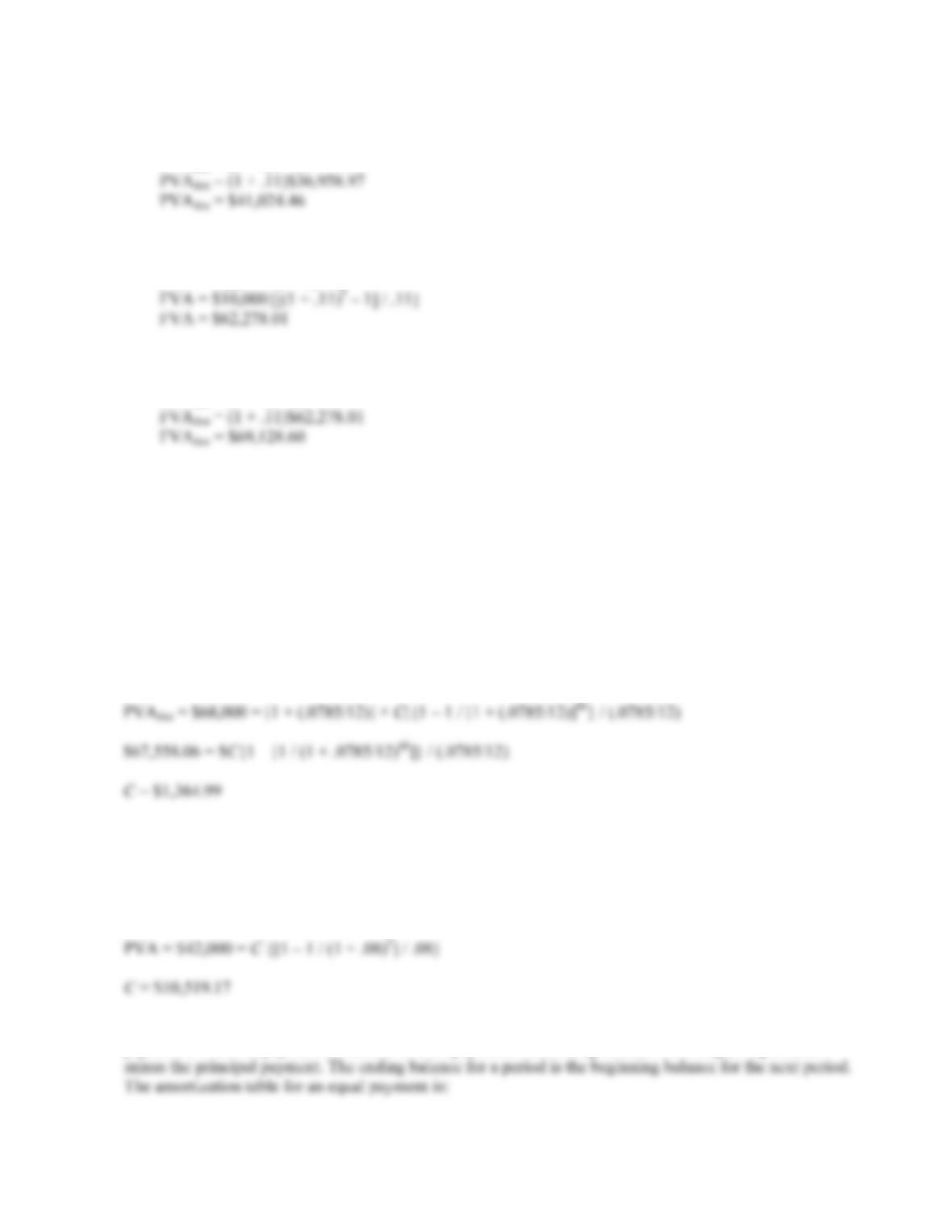CHAPTER 6 B-85
If the payments are an annuity due, the present value will be:
PVAdue = (1 + r) PVA
b. We can find the future value of the ordinary annuity as:
FVA = C{[(1 + r)t – 1] / r}
If the payments are an annuity due, the future value will be:
FVAdue = (1 + r) FVA
c. Assuming a positive interest rate, the present value of an annuity due will always be larger than the
present value of an ordinary annuity. Each cash flow in an annuity due is received one period earlier,
which means there is one period less to discount each cash flow. Assuming a positive interest rate, the
future value of an ordinary due will always higher than the future value of an ordinary annuity. Since
each cash flow is made one period sooner, each cash flow receives one extra period of compounding.
54. We need to use the PVA due equation, that is:
PVAdue = (1 + r) PVA
Using this equation:
Notice, when we find the payment for the PVA due, we simply discount the PV of the annuity due back
one period. We then use this value as the PV of an ordinary annuity.
55. The payment for a loan repaid with equal payments is the annuity payment with the loan value as the PV of
the annuity. So, the loan payment will be:
The interest payment is the beginning balance times the interest rate for the period, and the principal
payment is the total payment minus the interest payment. The ending balance is the beginning balance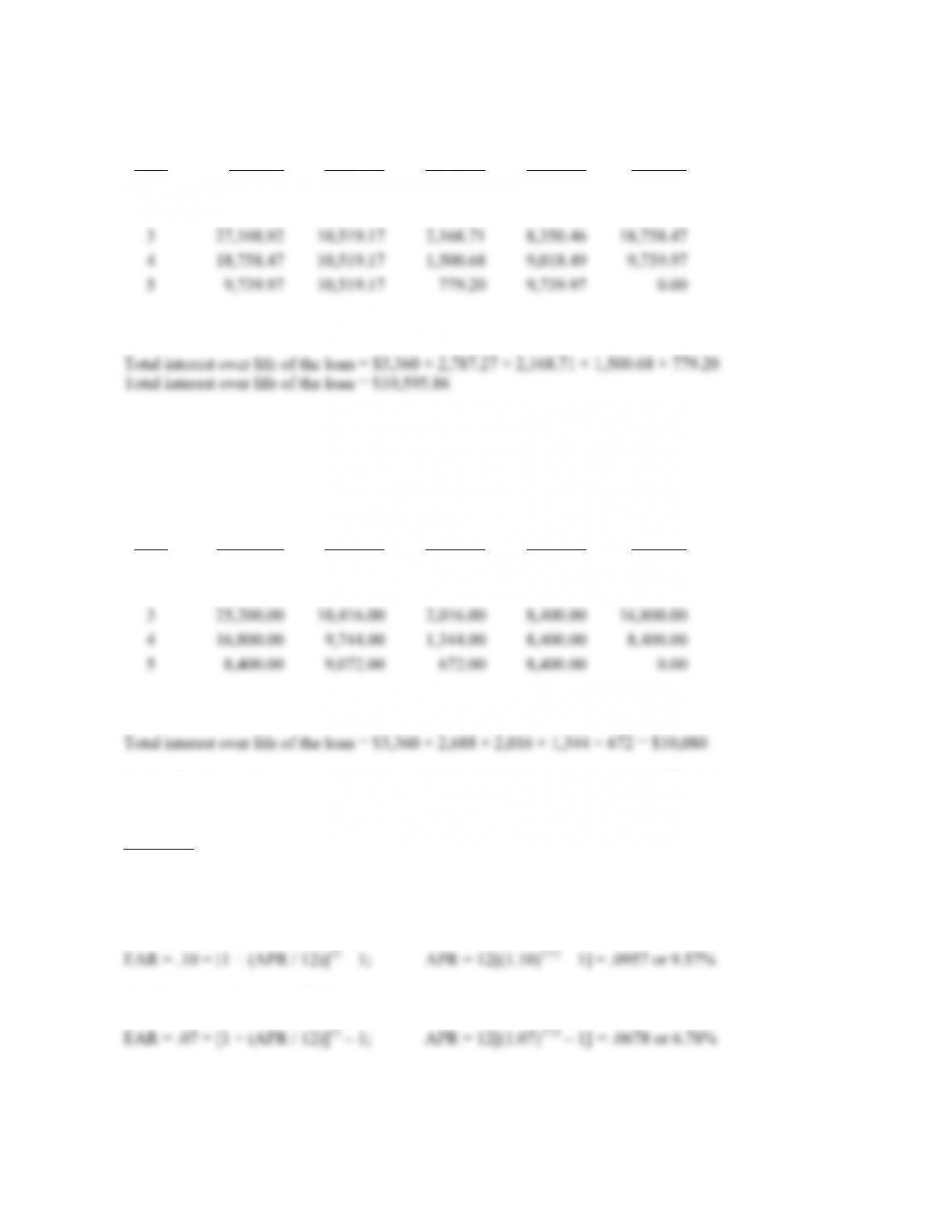B-86 SOLUTIONS
Year
Beginning
Balance
Total
Payment
Interest
Payment
Principal
Payment
Ending
Balance
1 \$42,000.00 \$10,519.17 \$3,360.00 \$7,159.17 \$34,840.83
2 34,840.83 10,519.17 2,787.27 7,731.90 27,108.92
In the third year, \$2,168.71 of interest is paid.
56. This amortization table calls for equal principal payments of \$8,400 per year. The interest payment is the
beginning balance times the interest rate for the period, and the total payment is the principal payment plus
the interest payment. The ending balance for a period is the beginning balance for the next period. The
amortization table for an equal principal reduction is:
Year
Beginning
Balance
Total
Payment
Interest
Payment
Principal
Payment
Ending
Balance
1 \$42,000.00 \$11,760.00 \$3,360.00 \$8,400.00 \$33,600.00
2 33,600.00 11,088.00 2,688.00 8,400.00 25,200.00
In the third year, \$2,016 of interest is paid.
Notice that the total payments for the equal principal reduction loan are lower. This is because more
principal is repaid early in the loan, which reduces the total interest expense over the life of the loan.
Challenge
57. The cash flows for this problem occur monthly, and the interest rate given is the EAR. Since the cash flows
occur monthly, we must get the effective monthly rate. One way to do this is to find the APR based on
monthly compounding, and then divide by 12. So, the pre-retirement APR is:
And the post-retirement APR is: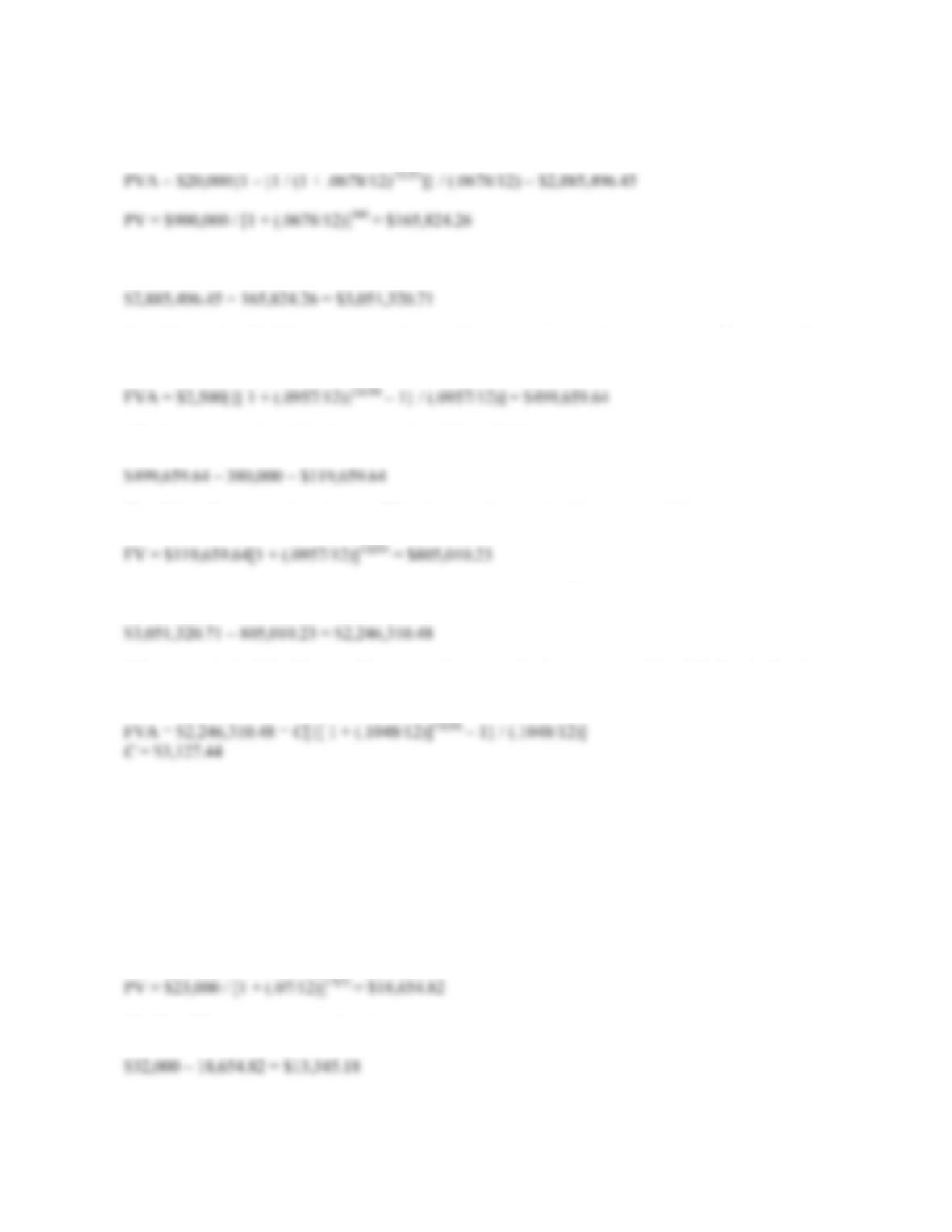CHAPTER 6 B-87
First, we will calculate how much he needs at retirement. The amount needed at retirement is the PV of the
monthly spending plus the PV of the inheritance. The PV of these two cash flows is:
So, at retirement, he needs:
He will be saving \$2,500 per month for the next 10 years until he purchases the cabin. The value of his
savings after 10 years will be:
After he purchases the cabin, the amount he will have left is:
He still has 20 years until retirement. When he is ready to retire, this amount will have grown to:
So, when he is ready to retire, based on his current savings, he will be short:
This amount is the FV of the monthly savings he must make between years 10 and 30. So, finding the
annuity payment using the FVA equation, we find his monthly savings will need to be:
58. To answer this question, we should find the PV of both options, and compare them. Since we are
purchasing the car, the lowest PV is the best option. The PV of the leasing is simply the PV of the lease
payments, plus the \$99. The interest rate we would use for the leasing option is the same as the interest rate
of the loan. The PV of leasing is:
PV = \$99 + \$450{1 – [1 / (1 + .07/12)12(3)]} / (.07/12) = \$14,672.91
The PV of purchasing the car is the current price of the car minus the PV of the resale price. The PV of the
resale price is:
The PV of the decision to purchase is: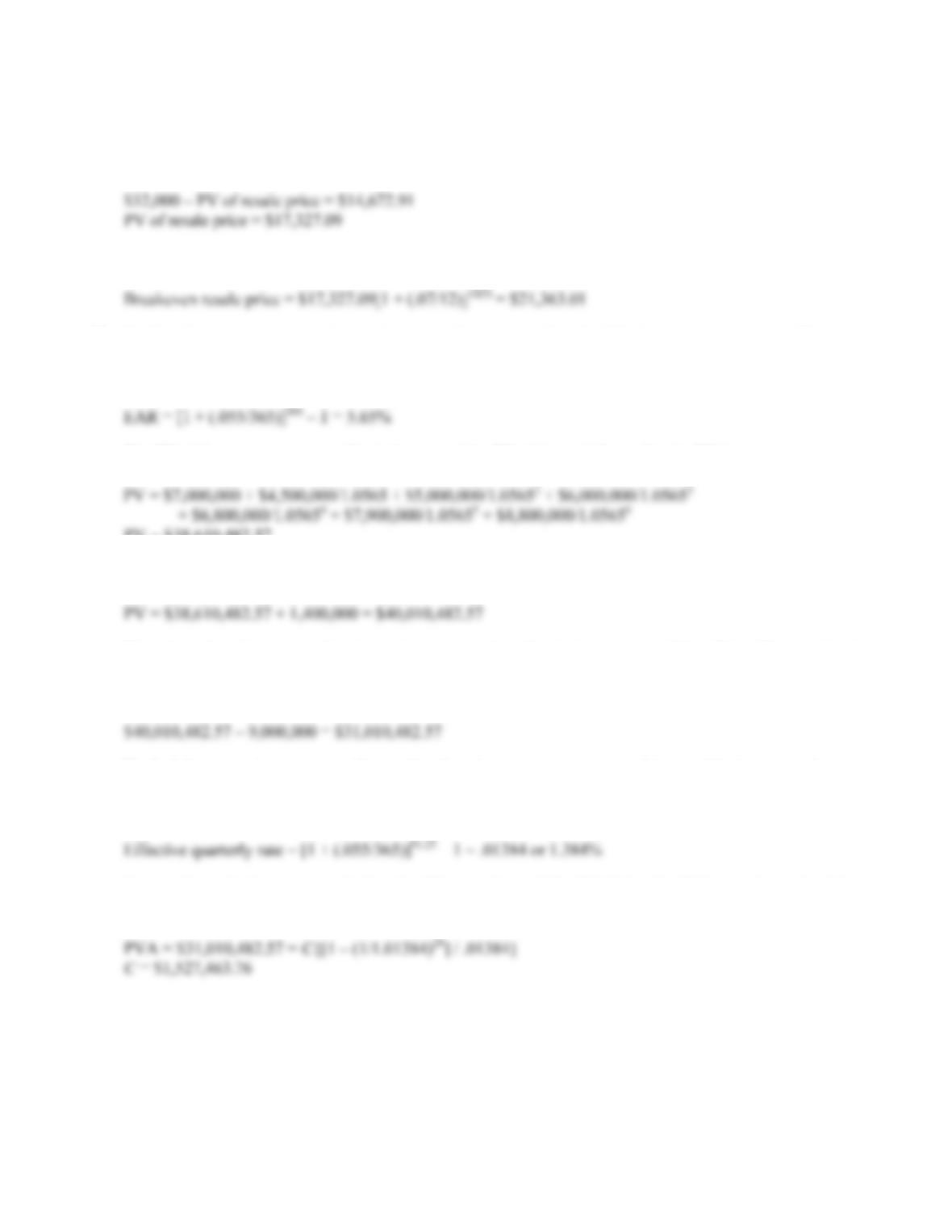B-88 SOLUTIONS
In this case, it is cheaper to buy the car than leasing it since the PV of the purchase cash flows is lower. To
find the breakeven resale price, we need to find the resale price that makes the PV of the two options the
same. In other words, the PV of the decision to buy should be:
The resale price that would make the PV of the lease versus buy decision is the FV of this value, so:
59. To find the quarterly salary for the player, we first need to find the PV of the current contract. The cash
flows for the contract are annual, and we are given a daily interest rate. We need to find the EAR so the
interest compounding is the same as the timing of the cash flows. The EAR is:
The PV of the current contract offer is the sum of the PV of the cash flows. So, the PV is:
PV = \$38,610,482.57
The player wants the contract increased in value by \$1,400,000, so the PV of the new contract will be:
The player has also requested a signing bonus payable today in the amount of \$9 million. We can simply
subtract this amount from the PV of the new contract. The remaining amount will be the PV of the future
quarterly paychecks.
To find the quarterly payments, first realize that the interest rate we need is the effective quarterly rate.
Using the daily interest rate, we can find the quarterly interest rate using the EAR equation, with the
number of days being 91.25, the number of days in a quarter (365 / 4). The effective quarterly rate is:
Now we have the interest rate, the length of the annuity, and the PV. Using the PVA equation and solving
for the payment, we get: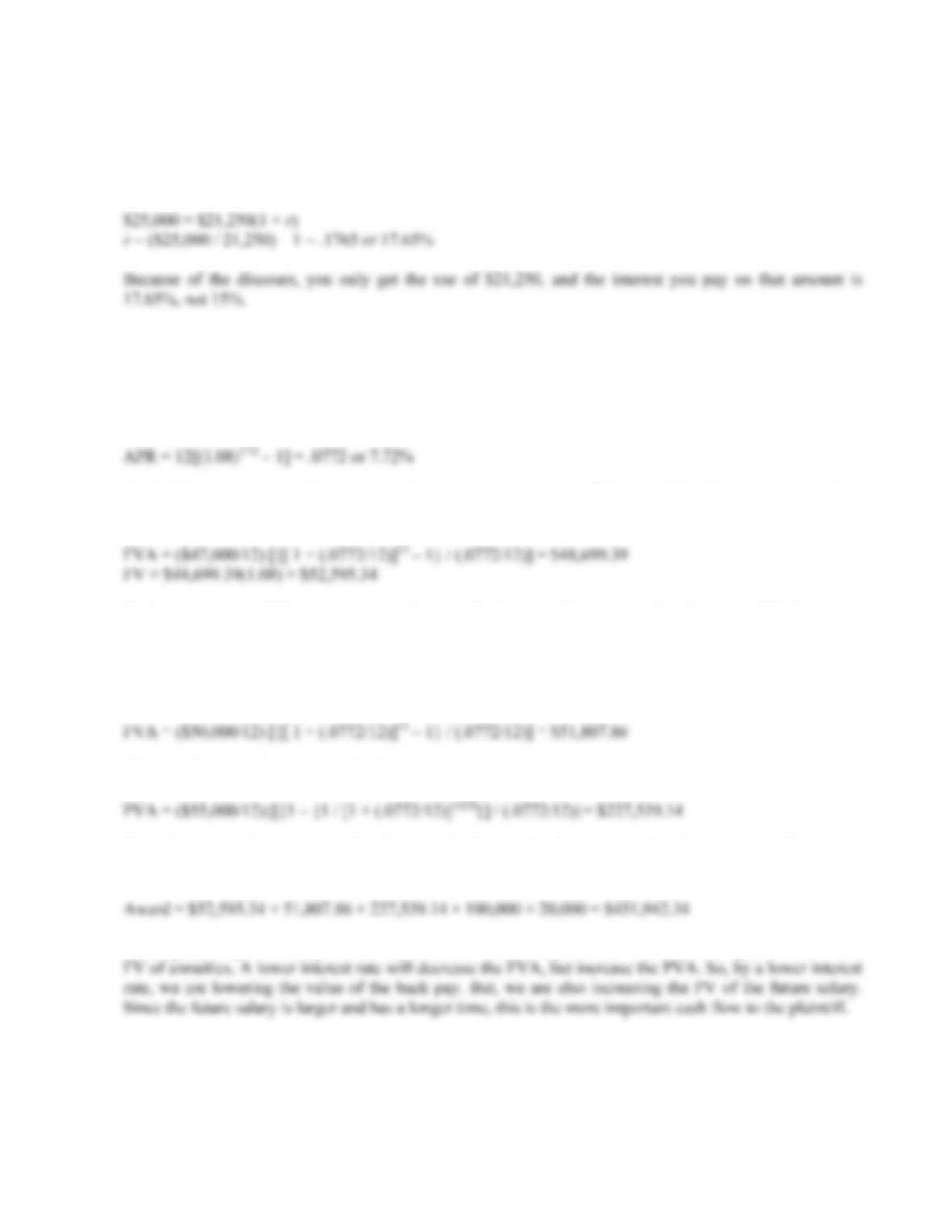CHAPTER 6 B-89
60. To find the APR and EAR, we need to use the actual cash flows of the loan. In other words, the interest rate
quoted in the problem is only relevant to determine the total interest under the terms given. The cash flows
of the loan are the \$25,000 you must repay in one year, and the \$21,250 you borrow today. The interest rate
of the loan is:
61. Here we have cash flows that would have occurred in the past and cash flows that would occur in the
future. We need to bring both cash flows to today. Before we calculate the value of the cash flows today,
we must adjust the interest rate so we have the effective monthly interest rate. Finding the APR with
monthly compounding and dividing by 12 will give us the effective monthly rate. The APR with monthly
compounding is:
To find the value today of the back pay from two years ago, we will find the FV of the annuity, and then
find the FV of the lump sum. Doing so gives us:
Notice we found the FV of the annuity with the effective monthly rate, and then found the FV of the lump
sum with the EAR. Alternatively, we could have found the FV of the lump sum with the effective monthly
rate as long as we used 12 periods. The answer would be the same either way.
Now, we need to find the value today of last year’s back pay:
Next, we find the value today of the five year’s future salary:
The value today of the jury award is the sum of salaries, plus the compensation for pain and suffering, and
court costs. The award should be for the amount of:
As the plaintiff, you would prefer a lower interest rate. In this problem, we are calculating both the PV and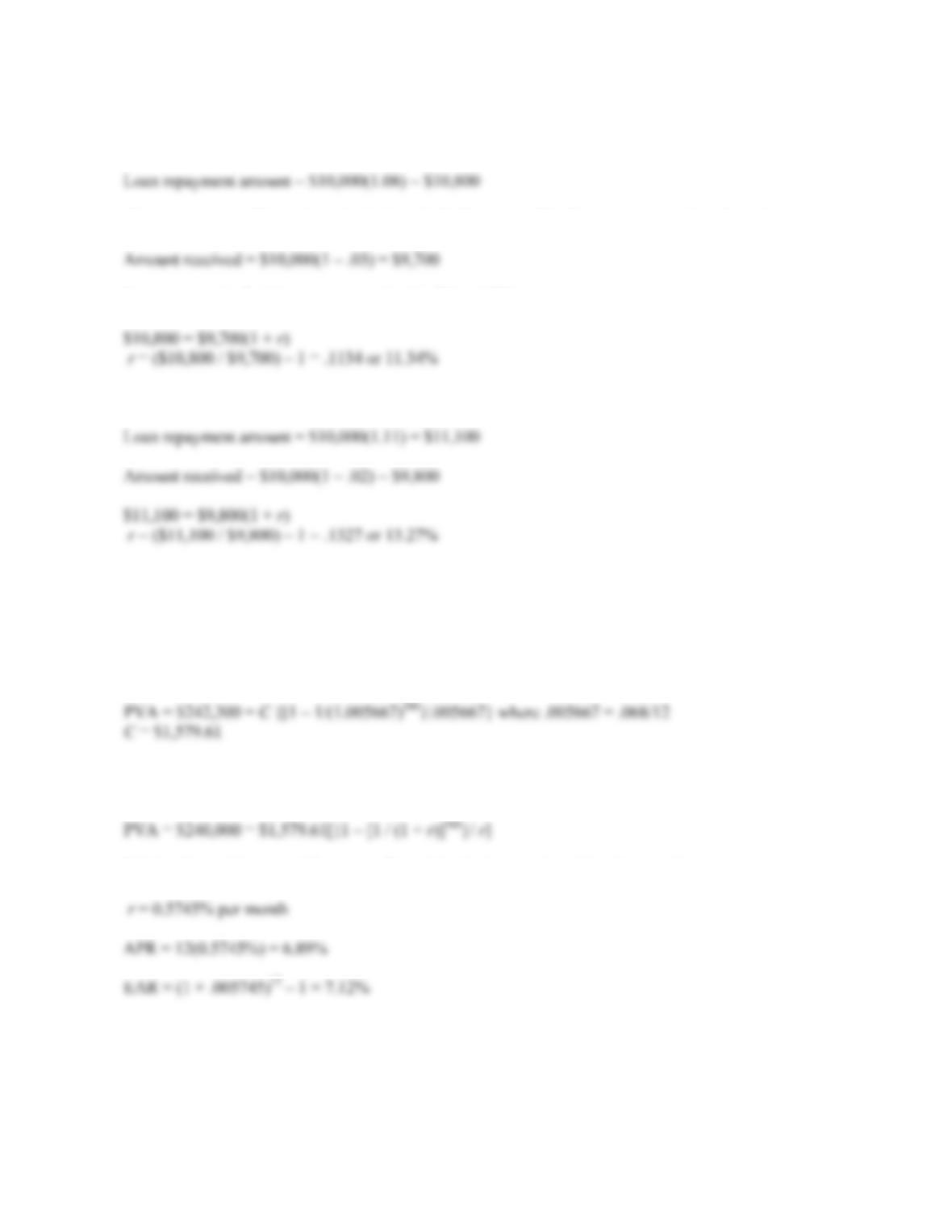B-90 SOLUTIONS
62. Again, to find the interest rate of a loan, we need to look at the cash flows of the loan. Since this loan is in
the form of a lump sum, the amount you will repay is the FV of the principal amount, which will be:
The amount you will receive today is the principal amount of the loan times one minus the points.
Now, we simply find the interest rate for this PV and FV.
63. This is the same question as before, with different values. So:
The effective rate is not affected by the loan amount since it drops out when solving for r.
64. First we will find the APR and EAR for the loan with the refundable fee. Remember, we need to use the
actual cash flows of the loan to find the interest rate. With the \$2,300 application fee, you will need to
borrow \$242,300 to have \$240,000 after deducting the fee. Solving for the payment under these
circumstances, we get:
We can now use this amount in the PVA equation with the original amount we wished to borrow,
\$240,000. Solving for r, we find:
Solving for r with a spreadsheet, on a financial calculator, or by trial and error, gives: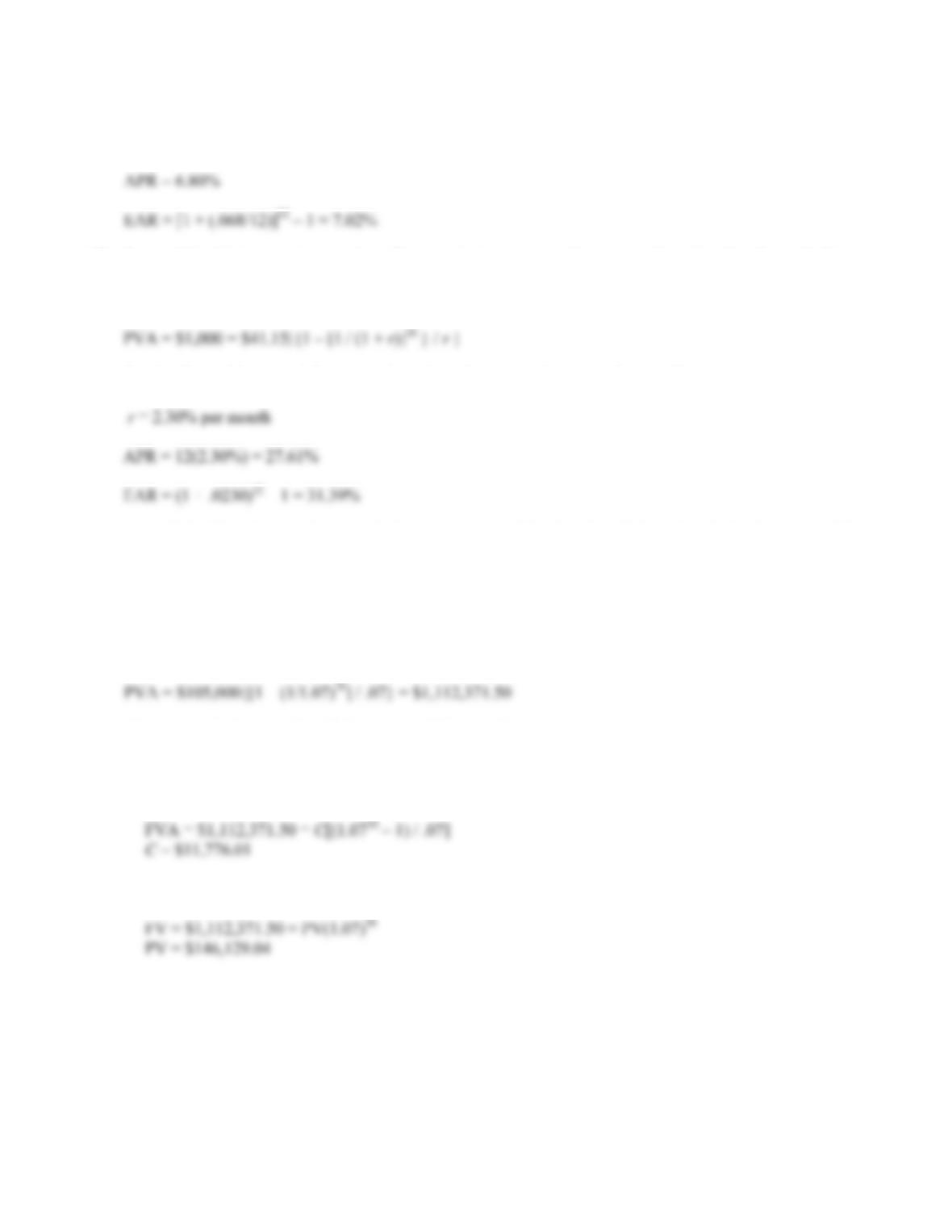CHAPTER 6 B-91
With the nonrefundable fee, the APR of the loan is simply the quoted APR since the fee is not
considered part of the loan. So:
65. Be careful of interest rate quotations. The actual interest rate of a loan is determined by the cash flows.
Here, we are told that the PV of the loan is \$1,000, and the payments are \$41.15 per month for three years,
so the interest rate on the loan is:
Solving for r with a spreadsheet, on a financial calculator, or by trial and error, gives:
It’s called add-on interest because the interest amount of the loan is added to the principal amount of the
loan before the loan payments are calculated.
66. Here we are solving a two-step time value of money problem. Each question asks for a different possible
cash flow to fund the same retirement plan. Each savings possibility has the same FV, that is, the PV of the
retirement spending when your friend is ready to retire. The amount needed when your friend is ready to
retire is:
This amount is the same for all three parts of this question.
a. If your friend makes equal annual deposits into the account, this is an annuity with the FVA equal to the
amount needed in retirement. The required savings each year will be:
b. Here we need to find a lump sum savings amount. Using the FV for a lump sum equation, we get: